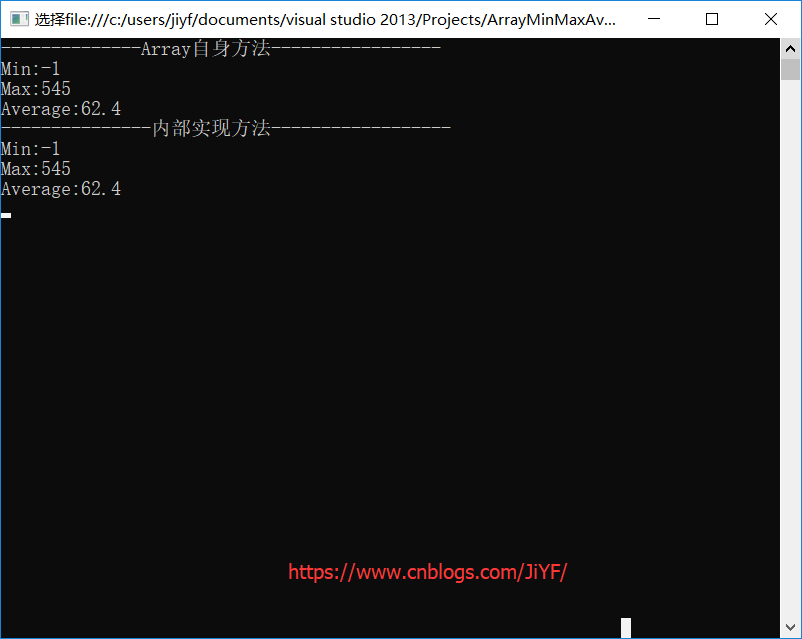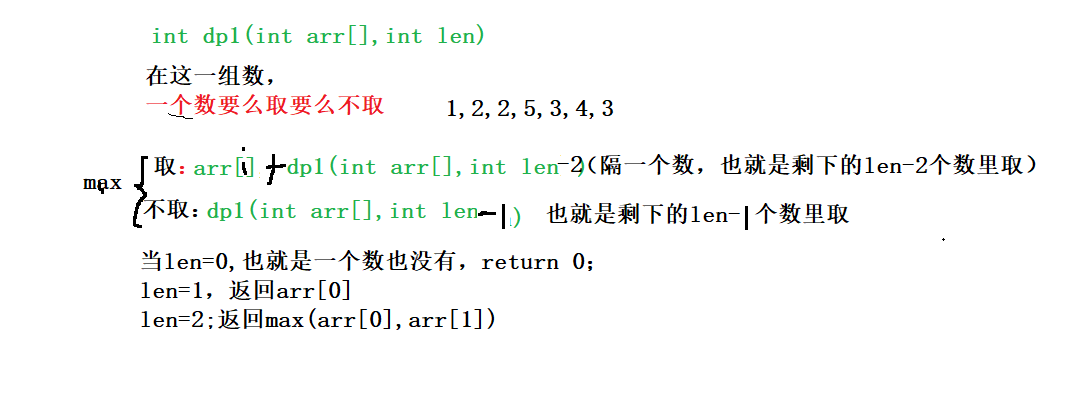• C#获取一个数组中最大值、最小值、平均值 1.给出一个数组 int[] array = new int[] { 1,2,4,3,0,-1,34,545,2,34}; 2.数组Array自带方法 本身是直接可以调用Min(),Max(),Average()方法来求出 最小值、最大值...
C#获取一个数组中的最大值、最小值、平均值
1.给出一个数组
 int[] array = new int[] { 1,2,4,3,0,-1,34,545,2,34};
2.数组Array自带方法
本身是直接可以调用Min(),Max(),Average()方法来求出 最小值、最大值、平均值
1             Console.WriteLine("--------------Array自身方法-----------------");
2             Console.WriteLine("Min:{0}",array.Min());
3             Console.WriteLine("Max:{0}", array.Max());
4             Console.WriteLine("Average:{0}", array.Average());
输出结果：
1 --------------Array自身方法-----------------
2 Min:-1
3 Max:545
4 Average:62.4
3.编码实现
最小值
        /// <summary>
/// 最小值
/// </summary>
/// <param name="array"></param>
/// <returns></returns>
public static int Min(int[] array)
{
if (array == null) throw new Exception("数组空异常");
int value = 0;
bool hasValue = false;
foreach (int x in array)
{
if (hasValue)
{
if (x < value) value = x;
}
else
{
value = x;
hasValue = true;
}
}
if (hasValue) return value;
throw new Exception("没找到");
}
最大值
        /// <summary>
/// 最大值
/// </summary>
/// <param name="array"></param>
/// <returns></returns>
public static int Max(int[] array)
{
if (array == null) throw new Exception("数组空异常");
int value = 0;
bool hasValue = false;
foreach (int x in array)
{
if (hasValue)
{
if (x > value)
value = x;
}
else
{
value = x;
hasValue = true;
}
}
if (hasValue) return value;
throw new Exception("没找到");
}
平均值
        /// <summary>
/// 平均值
/// </summary>
/// <param name="source"></param>
/// <returns></returns>
public static double? Average(int[] array)
{
if (array == null) throw new Exception("数组空异常");
long sum = 0;
long count = 0;
checked
{
foreach (int? v in array)
{
if (v != null)
{
sum += v.GetValueOrDefault();
count++;
}
}
}
if (count > 0) return (double)sum / count;
return null;
}
4.测试输出
测试代码
        static void Main(string[] args)
{
int[] array = new int[] { 1,2,4,3,0,-1,34,545,2,34};

Console.WriteLine("--------------Array自身方法-----------------");
Console.WriteLine("Min:{0}",array.Min());
Console.WriteLine("Max:{0}", array.Max());
Console.WriteLine("Average:{0}", array.Average());

Console.WriteLine("---------------内部实现方法------------------");
int min = Program.Min(array);
int max = Program.Max(array);
double? average = Program.Average(array);
Console.WriteLine("Min:" + min);
Console.WriteLine("Max:" + max);
Console.WriteLine("Average:" + average);
}
输出结果以上代码也是从.NET Framework中摘出来的，实际上 Array的自带求最大值、最小值、平均值的算法就是这样做的，在.NET Framework源码中可以看到
5.工程源码下载
源代码下载
展开全文最小值 平均值
• 功能：从一个字符数组中取出相应的整数、实数 作者：班草  */ #include #include #include void read_num( char *str , int *read_int , double *read_double);  int main() {  char *str = ...
/*

功能：从一个字符数组中取出相应的整数、实数

作者：班草
*/
#include <stdio.h>
#include <malloc.h>
#include <math.h>

int main()
{
char *str = NULL;

str = (char *)malloc(sizeof(char)*1024);
//分配堆空间

printf("input string:");
//输入字符串
scanf("%s",str);

//调用函数

int i = 0;

printf("int :");
//输出整型
for( i = 1 ; i <= read_int ; i ++ )
{
}
printf("\n");

printf("double:");
//输出实型
for( i = 1 ; i <= (int)read_double ; i++ )
{
}
printf("\n");

free(str);
//释放堆空间
str = NULL;

return 0;
}
{
int flag = 1;           //正负符号变量
int i_int = 1;          //整形数组的下标
int i_double = 1;       //实形数组的下标

int x = 0;
int u = 0;
int zi = 0;             //这三个变量用于将字符串数字转换为整形
double fzi = 0.0;       //存放小数点后的数值

while( *str != '\0' )   //遍历字符串
{
if( *str == '-' )   //判断是否为负号，
{
flag = -1;
str ++;
}

for( x = 0 ; *str >= '0' && *str <= '9' ; x++ , str ++ )  //读取字符串中一段连续的数字，转换为整型
{
//如125.6，读出为125
u = *str - '0';
if( x == 0 )
{
zi = u;
}
else
{
zi = zi * 10 + u;
}
}
if( *str == '.' && read == 1 )      //如果遇到小数点且小数点前为数字
{
str ++;
for( x = 0 ; *str >= '0' && *str <= '9' ; x++ , str ++ )
//读小数点后的值
{
u = *str - '0';
if( x == 0 )
{
fzi =(double)u / pow(10,x+1);
}
else
{
fzi += (double)u / pow(10,x+1);
}
}
if( fzi != 0.0 )
//如果小数点后读到了值，将其值转换为对应的实数然后加上前面的整数，存放到实型数组
{
read_double[i_double++] = (zi + fzi) * flag;
}
}
{
}
flag = 1;
}
}
展开全文C语言 字符串
• 1、 给定一个数组，可以从数组中取出下标不连续的任意个数，求可以取出的数的和的最大值， 例如：给出数组A[]={1,2,2,5,3,4,3}可以取出最大和为2+5+4=11。 现再给定数组{3,9,7,5,1,3,1,2,7}，能取出的数的和的...
1、 给定一个数组，可以从数组中取出下标不连续的任意个数，求可以取出的数的和的最大值，
例如：给出数组A[]={1,2,2,5,3,4,3}可以取出的最大和为2+5+4=11。现再给定数组{3,9,7,5,1,3,1,2,7}，能取出的数的和的最大值是24。
2、分析3、代码

private static int dp1(int arr[],int len) {
int ret=0;
if (len==0) {
return 0;
}else if (len==1) {
return arr;
}else if (len==2) {
return Math.max(arr,arr);
}else {
for(int i=2;i<len;i++) {
int r1=dp1(arr,len-2)+arr[i];
int r2=dp1(arr,len-1);
ret=Math.max(r1,r2);
}
return ret;

}
}

//优化
private static int dp1(int arr[]) {
int n = nums.length;
if (n == 0) {
return 0;
}
if (n == 1) {
return nums;
}
int[] dp = new int[n];
dp = nums;
dp = Math.max(nums, nums);
for (int i = 2; i < n; i++) {
dp[i] = Math.max(dp[i - 1], dp[i - 2] + nums[i]);
}
return dp[n - 1];
}

**
2. 给定一个正整数s, 判断一个数组arr中，是否有一组数字加起来等于s。
**
/**
*
* 2. 给定一个正整数s, 判断一个数组arr中，是否有一组数字加起来等于s。
*/
private static boolean isS(int[] arr,int i,int s) {
if (i==0) {
return arr==s;
}else if (s==0) {
return true;
}else if (arr[i]>s){
return isS(arr,i-1,s);

}else {
return isS(arr,i-1,s)||isS(arr,i-1,s-arr[i]);//又可分为两种情况，arr[i-1]>s
}
}
private static boolean isS2(int[] arr,int s) {
boolean set[][]=new boolean[arr.length][s+1];
set[arr]=true;
int i=0;
int j=0;
for (i = 0; i < arr.length ; i++) {
set[i]=true;
}
for (i = 1; i < arr.length; i++) {
for (j = 1; j < s+1; j++) {
if (arr[i]>j){
set[i][j]=set[i-1][j];
}else {
boolean a = set[i-1][j];
boolean b = set[i-1][s-arr[i]];
set[i][j]=a||b;
}
}

}
return set[i-1][j-1];
}

神奇的口袋：

有一个神奇的口袋，总的容积是40，用这个口袋可以变出一些物品，这些物品的总体积必须是40。John现在有n个想要得到的物品，每个物品的体积分别是a1，a2……an。John可以从这些物品中选择一些，如果选出的物体的总体积是40，那么利用这个神奇的口袋，John就可以得到这些物品。现在的问题是，John有多少种不同的选择物品的方式。
输入描述:
输入的第一行是正整数n (1 <= n <= 20)，表示不同的物品的数目。接下来的n行，每行有一个1到40之间的正整数，分别给出a1，a2……an的值。
输出描述:
输出不同的选择物品的方式的数目。
示例1 输入
3 20 20 20
输出
3

代码：
import java.util.Scanner;

public class Main{
static int[] arr=new int;
static int n;
private static int count(int v,int i) {
if(v==0) {
return 1;
}if(i==n||v<0) {
return 0;
}

return count(v-arr[i],i+1)+count(v,i+1);//选arr[i]和不选arr[i]

}
public static void main(String[] args) {
Scanner in=new Scanner(System.in);
n=in.nextInt();

for (int i = 0; i < n; i++) {
arr[i]=in.nextInt();
}
System.out.println(count(40,0));
}
}

展开全文• 现在获取数组中最大最小值用的越来越多了，于是乎我编了方法供大家使用。代码如下，若有问题可以与我联系，咱们一起学习一起进步。 代码如下: function getMaximin (arr,maximin) { if (maximin == “max”) { ...
• go数组练习：从一个整数数组获取最大整数，最小整数，总数以及平均值
需求：从一个整数数组中获取最大整数，最小整数，总数以及平均值
注意：
如果不对max、min赋初始值，则其默认为0，可能会出现没有比0大或没有比0小的情况导致出错，因此一般将max和min赋值为数组第一个元素值。for循环既可以使用i进行遍历，也可以利用rang进行遍历，用_接收无用参数。
代码
package main

import "fmt"

func main() {
a := int{14, 64, 37, 72, 75, 37}
max := a
min := a
sum := 0
// 不进行初始赋值的情况下，没有数据小于0，可能导致结果出错
//var max int
//var min int
//var sum int
//for _, value := range a {
//	if value > max {
//		max = value
//	}
//	if value < min {
//		min = value
//	}
//	sum+=value
//}
for i := 0; i < len(a); i++ {
if a[i] > max {
max = a[i]
}
if a[i] < min {
min = a[i]
}
sum += a[i]
}
avg := float32(sum/len(a))
fmt.Printf("数组a的最大值为：%d\n数组a的最小值为：%d\n"+
"数组a的总和为：%d\n数组a的平均值为%f\n", max, min, sum, avg)
}


运行结果：展开全文• 之前也遇到过类似的问题，如求n个数组任意选取一个元素的所有组合都是想起来比较简单，但是设计成算法却颇费周折。如数组为{1, 2, 3, 4, 5, 6}，那么中取出3个元素的组合有哪些，取出4个元素的组...算法
• 从一个字符数组中读出相应的整数、实数// author：yangyang。 //main.c #include "stdafx.h" void shishupanduan(int flag); char s; char *p = s; void main() { gets_s(s); char zhengshu; int i = 0; ...
• 数组中一个或连 * 续的多个整数组成一个数组。求所有子数组的和的最大值。要求时间复杂度为O(n)。 * * @param arr 输入数组 * @return 最大的连续子数组和 */ public static int ...
• 主要介绍了c#获取数组中最大数的值,需要的朋友可以参考下
• ② 在源数组中索引为0的数组元素开始，拷贝index个数组元素到另外一个目标数组 ③ 把临时变量temp赋值给目标数组索引为0的位置 代码如下:public static class ArrHelper {  ///  /// 对类型为T的数组
• 2. 请用户输入一个整数1--7的值： 根据用户输入，从数组中取出对应的星期名称，例如： 用户输入：1 程序提示：星期一 3. 为了防止用户输入小于1或者大于7的值，请使用异常处理从数组中取出对应的“星期名称”，...java
• 这里先讲种类似于快速排序的方法。注意题目要求，不要求完全排序，只要求最快解决问题！...原话是1亿数据里，找出前个最大的。 首先看源码吧： void findMaxN（int a[], int start, in
• 代码： <!... <title>Title ... var items = ['1','4','9','10'];... var item = items[Math.floor(Math.random()*items...floor() 方法返回小于等于x的最大整数。 eg: console.log(Math.floor(1.6)); //结果为1javascript
• //从一个整数数组中取出最大整数，最小整数，总和，平均值 //声明一个int类型的数组，并且随意的赋初值 int[] nums={1,2,3,4,5,6,7,8,9,0}; //声明两个变量用来存储最大值和最小值 int max=int.MinValue;//nums...
• array_column() - 从数组中取出一
• 给定一个数组[1,2,3,4,5,6,7,8,9,10],求出数组中任意组合为之和等于10的数组。 注意，每一种组合中一个数只能出现一次。 数组共有N个元素，那么我们可以用1到2^N的二进制来求解，若二进制数该位置是1,则将其取出求和...
• #!/usr/bin/python # -*- coding:utf-8 -*- import random def randomText(textArr): length = len(textArr) ...randStr = randomText(['数字','数字二','数字三','数字4','数字5']) print randStr
• 问题描述 我想得到的是特定数，可能不是连续的，而且 数是取出的。 代码 private static int[] randomCreate(int ...//创造一个用于接收的数组 for (int i = 0; i &lt; rands.length; i++) { int temp ...
• 从数组中取出任意数，求和为指定值的解 PHP <?php $cc = array(1,9,34,56,10,7,3,28,9); function demo($cc,$val){ for ($i = 1; $i < 1 << count($cc); $i++) {$sum = 0; $temp = ""; ...php 算法 • //获取数组中最大值 var nums=[5,13,20,18,32] //声明变量用于保存最大值，吧第一个元素作为最大值 //在比较的时候可以第二个元素开始比较 var s=nums; //遍历数组，得到每个元素 for(var i=0;i<nums.length...js javascript • 方法： if ($row) { $data = array();$arr = $row;$discrepancyValue = $abnormalValue = 0; //最小值 do { foreach ($row as $ke =&gt;$va) { switch (coun...
• 假定大数组包含了n个小数组，分别找到每个小数组最大值，然后把它们串联起来，形成一个数组. 我们可以用for循环来迭代数组，并通过arr[i]的方式来访问数组的每个元素。 得到数组中最大值我们可以使用Math....JavaScript 算法
• 读取一个包含10个元素的数组，并在其中找到两个最大元素，然后打印它们的总和。 #include <stdio.h> int main(){ int Max,max; const int N = 10; int num[N]; for (int i = 0; i<N; i++) { scanf("%d...算法
• 可以使用numpy的random....#a为数组或int值，为数组时会数组中随机选择元素，为int值时会先生成一np.arange(a)的数组，然后从中随机选择元素 #size为int值，为选择元素的个数 #replace默认为Tru...python numpy
• int [] arr = {1,2,3,4}; //产生0-(arr.length-1)的整数值,也是数组的索引 int index=(int)(Math.random()*arr.length); int rand = arr[index];
• 题目： 输入一个整数数组，将它们连接起来排成一个数，输出能排出的所有数字最小的一个。 例如输入数组{122,12,123}，则输出这两个能排成的最小数字12122123。 思路： 新排序规则：从整数数组中取出两个数a和b,...
• 最大值获取：从数组的所有元素找出最大值。 实现思路： 定义变量，保存数组0索引上的元素 遍历数组，获取出数组中的每元素 将遍历到的元素和保存数组0索引上值的变量进行比较 如果数组元素的值大于了变量的...
• def func(arr,d): l = [] 注释：枚举 for index,i in enumerate(arr): 注释：遍历索引 for j in range(index+1): if i + arr[j] == d: if i != arr[j]: l.append((i,arr(j))) return l
• 这是一个呆萌炫酷吊炸天的前端算法题，曾经乃至现在也是叱咤风云在各个面试场景。 可以这样说，有 90% 以上的前端工程师不会做这个题目。 这道题涉及的知识点很多，虽然网上也有相关的解答文章，但是在算法小分队......